# Lists in Scala

Learn what lists are and how they can be leveraged in the Scala Programming Language.
Oct 2019  · 6 min read

You may also be interested in reading the following tutorials:

## Introduction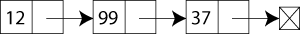Source: Wikipedia - List (abstract data type)

A singly linked list structure, implementing a list with 3 integer elements (Source: Wikipedia - List (abstract data type)).

A list, in general, is a sequence of abstract data types that represents a fixed number of values. Values can be manipulated programmatically by a program and can also occur more than once in a list. An instance of a list is shown in the above figure as a singly linked list consisting of three integer values. The list is also considered as a data structure, and one such example of a list as a data structure is linked lists.

Many programming languages provide support for lists, and one such example wherein people use lists extensively is Python. A list can often be constructed by writing the items in a sequence, separated by commas, semicolons, or spaces, within a pair of delimiters such as parentheses `()`, brackets `[]`, braces `{}`, or angle brackets `<>`. Some languages like Python may allow list types to be indexed or sliced like array types, in which case the data type is more accurately described as an array (Source: Wikipedia - List (abstract data type)).

Specific to Scala, a list is a collection which contains `immutable` data, which means that once the list is created, then it can not be altered. In Scala, the list represents a linked list.

In a Scala list, each element need not be of the same data type. The implementation of Scala lists uses a mutable state internally during the construction phase. In Scala, the list is defined under the `scala.collection.immutable` package and hence, they are immutable. Finally, a Scala List has various methods like `add`, `prepend`, `max`, `min`, etc.

#### Syntax for defining a Scala List

val variable_name: List[type] = List(item1, item2, item3) or val variable_name = List(item1, item2, item3) A list in Scala is mostly like a Scala array. However, the Scala List is immutable and represents a linked list data structure. On the other hand, Scala array is flat and mutable.

Let us quickly understand the above difference with the help of an example.

#### Scala List vs. Scala Array

Let's first define an array in scala.

Then you will modify a value at the 1st index since scala arrays are zero-indexed and see if you can update it.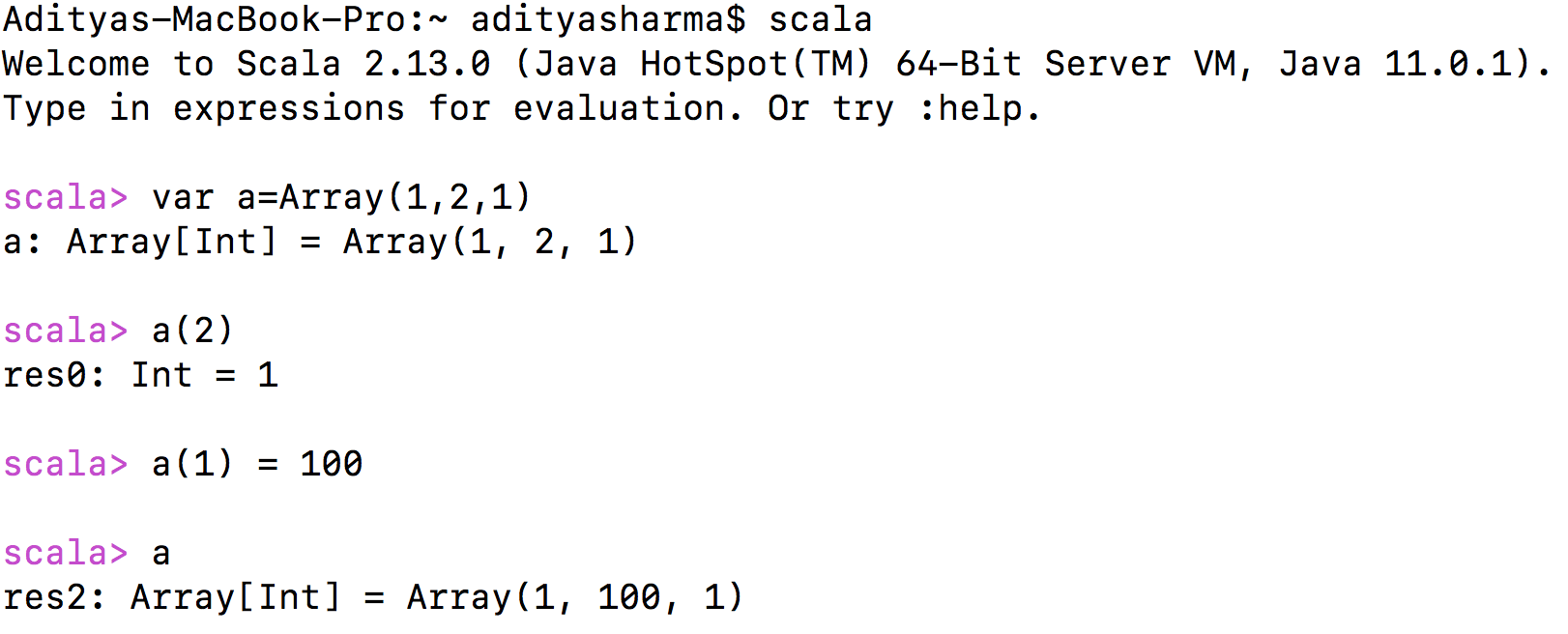So, as you can see from the above snippet of code, the scala array did allow you to modify the value at the 1st index.

Now, you will define a `Scala List` and try to mimic the same as above.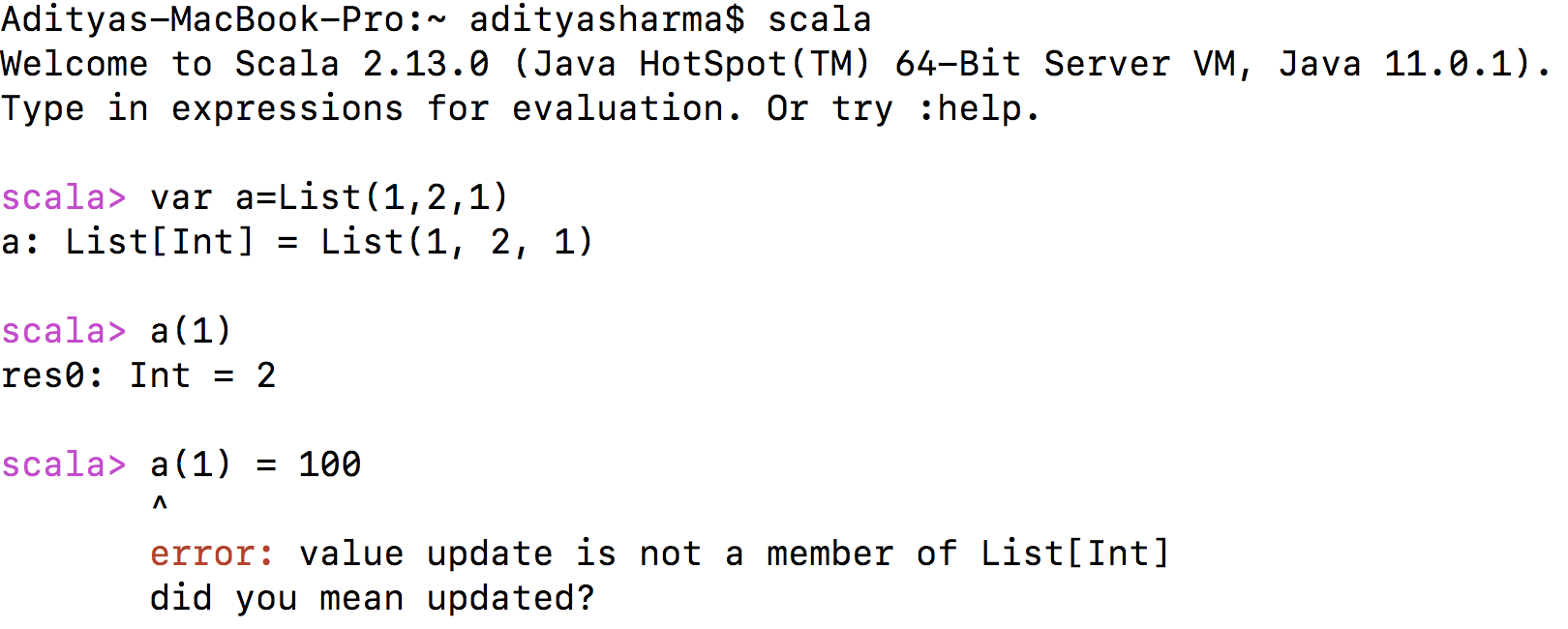Well, from the above example, you can conclude that since the Scala List's are immutable, updating the value resulted in an error.

### Scala List Methods

• head: This method returns the first element of the scala list.

• tail: This method returns all the elements except the first.

• isEmpty: This method checks if the list is empty in which case it returns True else False.

• fill(): This method creates a uniform list consisting of zero or more copies of the specified element.

• reverse: This method reverses the element of the list.

• \$:::\$ or \$.:::\$ or .concat(): Concatenates two or more lists.

• tabulate(): This method can tabulate a list. It's similar in spirit to `fill()` method, but unlike the `fill()` method, it takes two arguments. The first argument gives the dimensions of the list to create while the second describes the elements of the list, which are computed from a function.

Do not worry if some of the above methods do not make much sense to you, since you will be implementing them in Scala with the help of an example but before that let's learn how to define Scala Lists.

You will create Scala List with `strings`, `integers (both 1D & 2D)` data types. You will also learn to create a list consisting of both strings and integers.

#### 1-D Integer List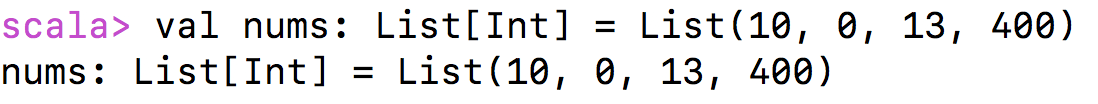#### 1-D List with both Integer and String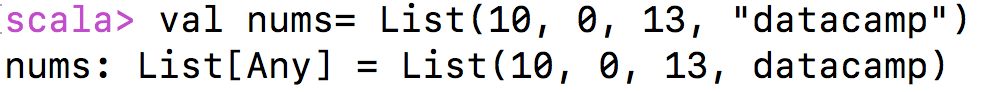#### 1-D String List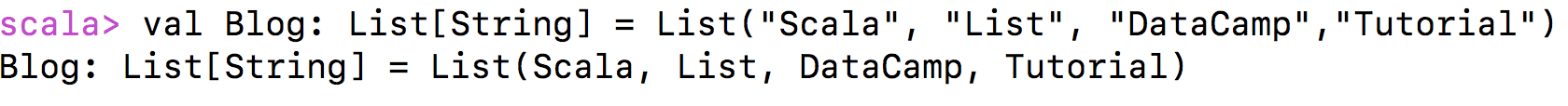#### 2-D List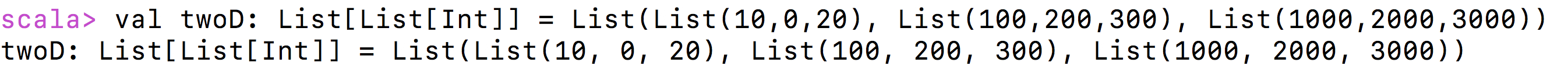### Arithmetic Operation on Lists

In this example, you will be making use of lists instead of defining just variables. Unlike a variable, a List can hold n number of values; you will be performing the arithmetic operations on the two lists instead of two variables.

So, let's quickly learn how it can be done.

For you to apply the arithmetic operations, you will be making use of the `zipped.map` function which will be followed by the lists x and y. You will then specify in the `zipped.map` method the operation you would like to perform as an argument.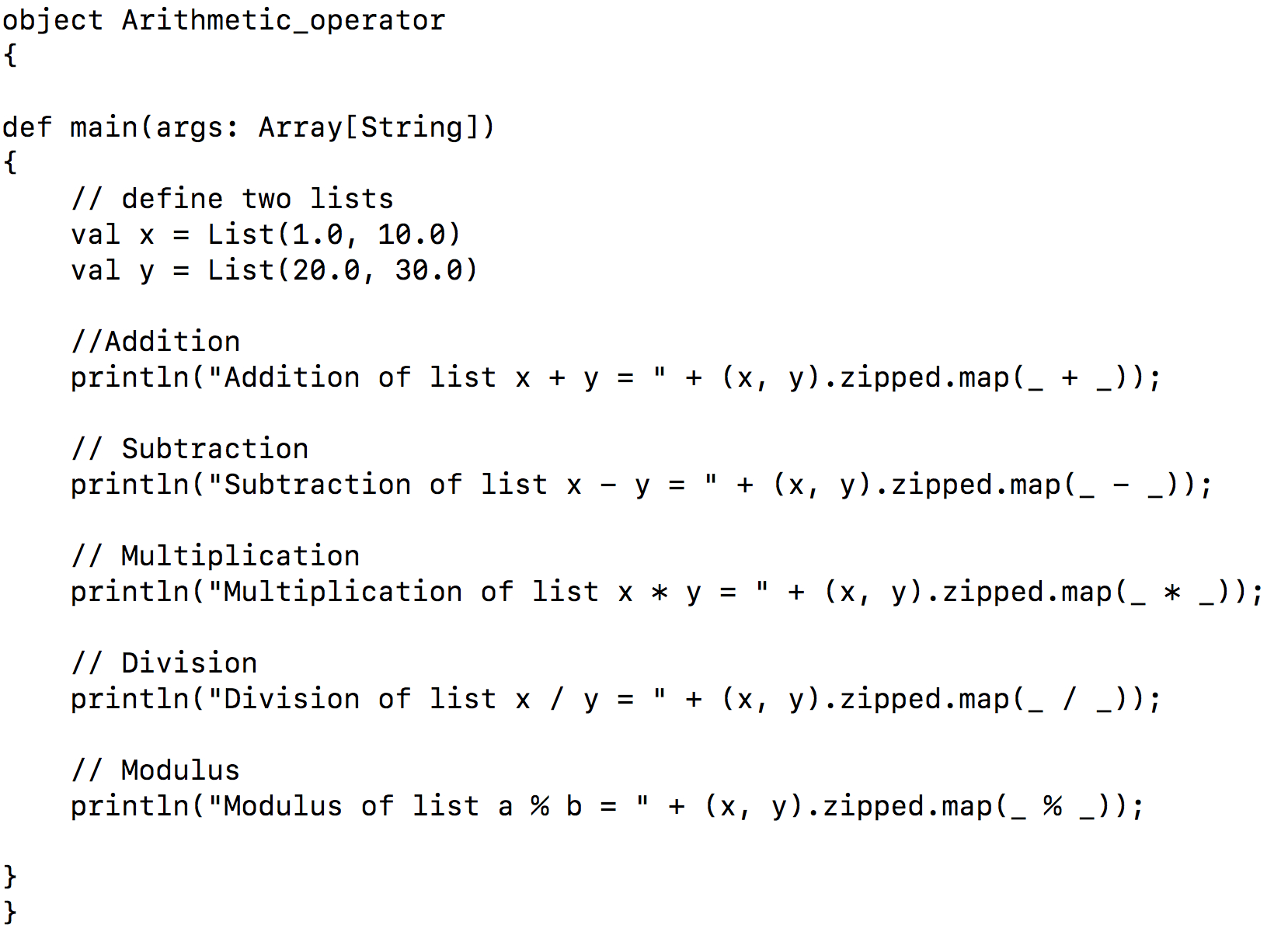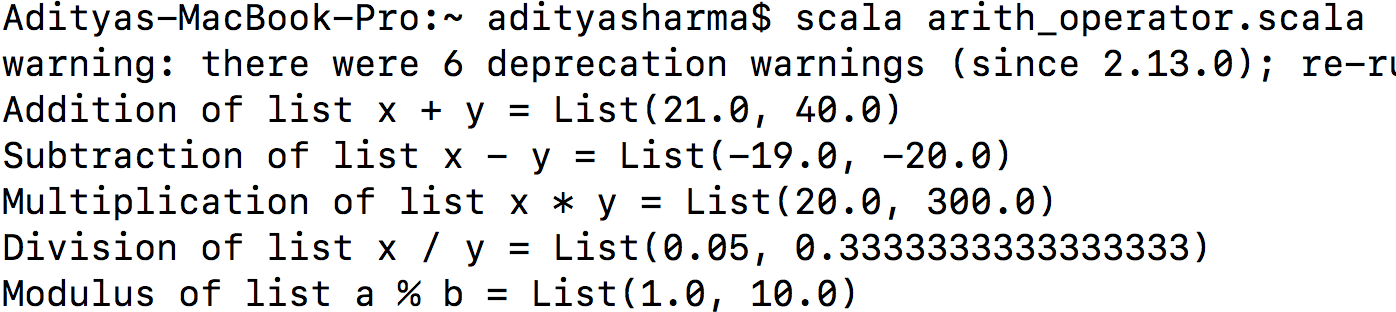Finally, let's write a code which will have all of these methods.

``````object Scala_List_Methods
{

def main(args: Array[String])
{
// define two lists
val x = List(1, 40, 10)
val y = List(20, 25, 30)
val z = List("DataCamp", "Tutorials", "Are", "The", "Best")

println();
println();

//Tail
println("Tail of list x, y, z = " + (x.tail, y.tail, z.tail));
println();

//isEmpty
println("Is list x, y, z empty? = " + (x.isEmpty, y.isEmpty, z.isEmpty));
println();

//Concatenate
println("Concatenating x, y, z lists with the first method = " + (x:::y:::z));
println("Concatenating x, y, z lists with the second method = " + ((x).:::(y).:::(z)));
println("Concatenating x, y, z lists with the third method = " + (List.concat(x,y,z)));
println();

//Reverse
println("Reverse of x, y, z is = " + (x.reverse, y.reverse, z.reverse));
println();

//Fill
println("Output of Fill method is = " + (List.fill(5)(z)));
println();

//Tabulate
println("Output of Tabulate method is = " + (List.tabulate(5)(x => x * x * x)));
}
}
``````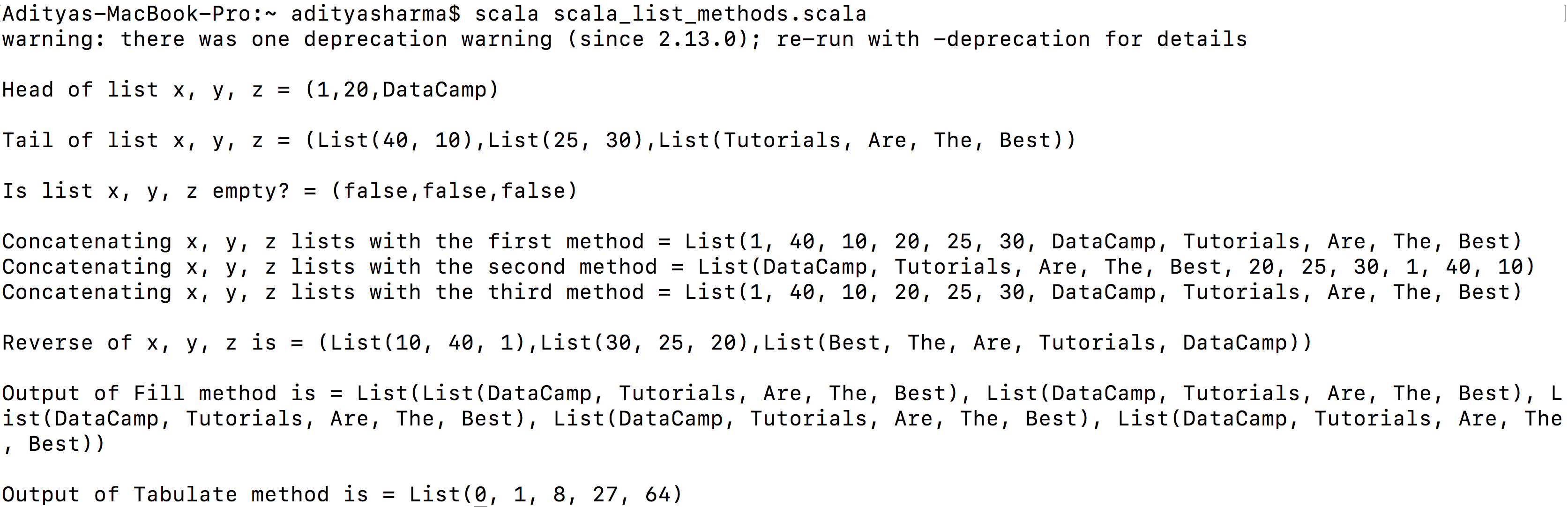## Conclusion

Congratulations on completing this Scala tutorial.

Please feel free to ask any questions related to this tutorial in the comments section below.

### Introduction to Scala

Beginner
3 hours
18,804
Begin your journey with Scala, a popular language for scalable applications and data engineering infrastructure.
See Details
See More